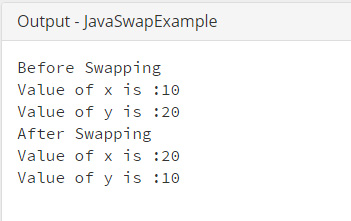# Java Program to Swapping Two Numbers Using a Temporary Variable

This Java program is used to demonstrates swapping two numbers, using a temporary variable.

Example:
``````public class JavaSwapExample {

public static void main(String[] args) {
int x = 10;
int y = 20;

System.out.println("Before Swapping");
System.out.println("Value of x is :" + x);
System.out.println("Value of y is :" + y);

//swap the value
swap(x, y);
}

private static void swap(int x, int y) {
int temp = x;
x = y;
y = temp;

System.out.println("After Swapping");
System.out.println("Value of x is :" + x);
System.out.println("Value of y is :" + y);
}
}
``````
Program Output:Explanation:
In this program, a class name JavaSwapExample is being declared which contains the main() method. Inside the main(), two integer type variables are declared name x and y and are initialized with values 10 and 20 respectively.

Now in this program, you have to swap the value that is present in x to y and that of y in x, i.e. after swapping the current value of 'x' and 'y', the 'x' will store 20 and 'y' will store 10. The statements:

System.out.println("Value of x is :" + x);

System.out.println("Value of y is :" +y);

Print the current value of x and y. Then the swap() user defined function is called which is having 2 parameters x and y. The two parameters are passed. The user defined function swap() is defined next, where the actual swapping is taking place.

private static void swap(int x, int y)

Since the swapping is done using the third variable, here you will include another integer type variable name temp where you first put the value of 'x', the in 'x' put the value of 'y' and then from temp, initialize the value of y as done above -

y = temp;

The two statements:

System.out.println("Value of x is :" + x);

System.out.println("Value of y is :" +y);

Prints the value after  swapping.